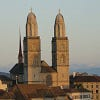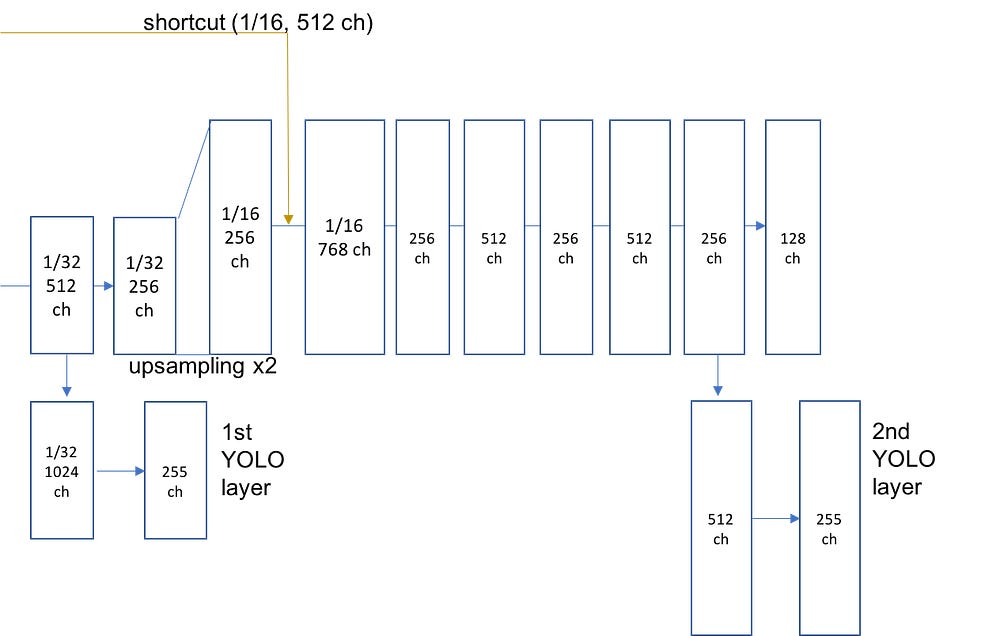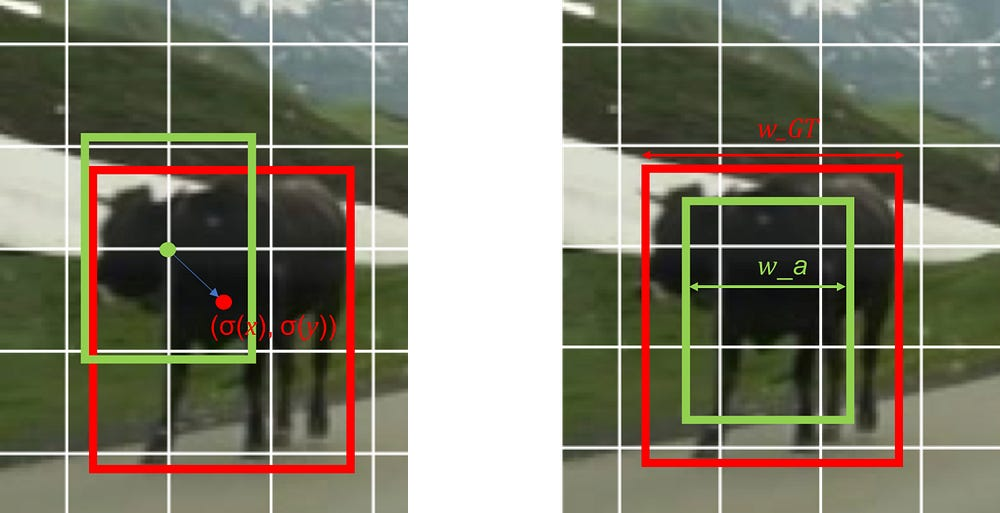# Reproducing training performance of YOLOv3 in PyTorch (Part1)

Source: Deep Learning on Medium

### Part 1 : Network architecture and channel elements of YOLO layers

Hi, I’m Hiroto Honda, an R&D engineer at DeNA Co., Ltd. in Japan.

In this article, I share the details for training the detector, which are implemented in our PyTorch_YOLOv3 repo that was open-sourced by DeNA on Dec. 6, 2018.
Last time I introduced our repo and emphasized why it is important to reproduce training performance. This time, I would like to show the structure of the YOLOv3 network architecture and the channel elements of YOLO layers (detection layers) for multi-scale object detection. Some of the important details in this post are not written in the paper, but in the original implementation.

1. Network Architecture

YOLOv3 consists of the backbone network called darknet53, the upsampling network, and the detection layers called YOLO layers.

• Backbone Network : darknet53

The backbone network extracts the feature map from the input image. The network mainly adopts residual blocks as the basic components. Each residual block consists of a 3 × 3 and 1 × 1 convolutional layer pair with a shortcut connection. The total number of convolutional layers is 53, which is why the network is coined darknet53. The dimension of the final feature map has 1/32 times smaller spatial resolution than the input image.

• Upsampling network and YOLO layers

Three YOLO layers that are responsible for detecting objects at different scales are branched from the ‘upsampling network’ — the right-hand pyramid shape of Fig. 1. The details of the network are shown in Fig. 2.

At the first YOLO layer the grid resolution is 1/32 of the input image and large objects are detected. The final YOLO layer’s resolution is 1/8 and the layer is capable of detecting small objects. As shown in Fig. 2, there are several convolution layers and an upsampling layer between the YOLO layers. Each layer has the sub-layers of convolution, batch normalization and leaky ReLU activation. There are shortcut connections which concatenate the intermediate layers of darknet53 to the layer right after the upsampling layer.

The three-scale YOLO layers are adopted in YOLOv3. The benefit mainly is that the detector is capable of capturing smaller objects than when using only one YOLO layer without upsampling.Fig. 2 Upsampling network and YOLO layers. The rectangles stand for feature maps.

2. Channel Elements of YOLO layers (YOLO channel elements)

Each YOLO layer has a dimension of (f_h, f_w, ch), where f_h, f_w, and ch are the height, width, and number of channels of the feature map respectively.

The number of channels in each YOLO layer is N_anchor × (N_class +5), where N_anchor is the number of anchors and N_class the number of object classes. Fig. 3 shows the map of all the YOLO channel elements. By default, YOLOv3 uses 3 anchors and 80 classes. Therefore, the number of channels in one YOLO layer is 3 × (80 + 5).

The nine anchors are depicted in Fig. 3 using the actual aspect ratios. The anchor boxes are the dataset-dependent reference bounding boxes which are pre-determined using k-means clustering. There is clearly large variation in the anchor size — the largest anchor size is (373, 326) pixels and the smallest one (10, 13) pixels. The similar anchor to the target object’s bounding box has an advantage of being adjusted to the target more precisely than the others.

Now, how does each channel element work?

• x, y, w, h channels

The feature maps have 32 , 16, and 8 times as coarse resolution as the input image at three YOLO layers respectively. Each feature grid has the information that represents the coordinates of the detected bounding boxes.

Fig. 4 depicts the relationship between the anchor box and the ground-truth box. The centers of anchor boxes are defined to be at the top-left corner of the grid square. The values x, y at the x, y channels are trained so that displacement of the ground-truth box from the corner is inferred as the relative position within the grid box : (σ(x), σ(y)), where σ is sigmoid function whose output values range from 0 to 1 — w, h channel values w, h are used for adjusting the box width and height. As shown in Fig. 4 (right), w is trained so that the ratio between the anchor width w_a and the ground truth box width w_GT is exp(w).Fig. 4 Bounding box localization. The green box is an anchor box and the red box is a ground truth.
• obj channels

Values of obj channels represents ‘objectness’, which indicates the likelihood that an object belongs to the grid. If the obj value is 0.98 for instance, an object is more than likely there. Obj values are used to filter out the inferred objects with low likelihood.

• cls channels

The cls values are used for object classification. The number of class channels corresponds to the number of classes of the dataset — 80 in case of the COCO dataset. The channels are trained so that only the value of the class channel corresponding to the ground-truth class becomes 1 and the others 0. At inference time, you can just argmax the channel values to pick the channel index.

Summary:

• YOLOv3 detects objects of different sizes at three YOLO layers
• Each YOLO layer has grids of different resolution and three anchors with different shapes
• Each anchor of one grid has the following information: box center location, box size, objectness likelihood, and class probability

That’s it for this time. Next time, I am going to explain the most important part — target assignment to YOLO channels.

Part 0. Introduction

Part 1. Network Architecture and channel elements of YOLO layers

Part 2. How to assign targets to multi-scale anchors

Part 3. What are the actual loss functions?

Check out our PyTorch implementation of YOLOv3!!

https://github.com/DeNA/PyTorch_YOLOv3

Thank you, see you again in Part 2!